Mobile QR Code1. (Department of Electronics Engineering, Chungnam National University, Daejeon, 34134, Korea )

NAND flash memory, charge migration, reliability, retention, test pattern, activation energy

## I. INTRODUCTION

With the advent of the fourth industrial revolution, industries such as artificial intelligence ($A.I.$), the Internet of things ($IoT$), self-driving cars and big data are emerging. As this new industry appears, it leads to mass of data distribution and large capacity contents consumption. In accordance with this demand, memory capacity increases and the need for server investment grows (1,2). In order to satisfy this, research on high-performance 3D NAND flash memory is being actively conducted. High-performance memory requires high degree of integration, low bit cost, and low inter-cell interference. The 3D NAND flash memory can satisfy this demand (3,4).

Fig. 1. Flash memory structure and representation of the charge migration in (a) 2D planar structure, (b) 3D vertical structure.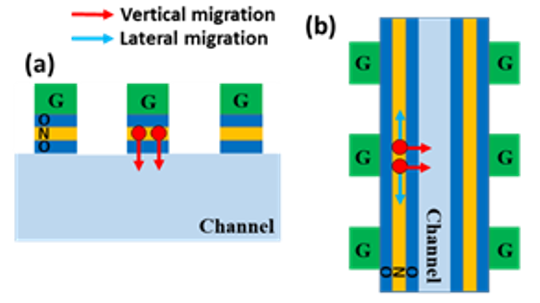As degree of integration increases, problems arise in physical ($degradation$ $of$ $lithography$ $pattern$), electrical ($shoot$ $channel$ $effect,$ $gate$ $induced$ $drain$ $leakage$), and reliability aspects ($retention$ $decrease$ $due$ $to$ $electronic$ $loss$). In particular, the degradation problem of retention caused not only vertical charge loss in the 2D structure but also other problems as the structure of the memory changed to 3D structure.

As shown in Fig. 1, the 3D structure shares the ONO layer. As a result, charge migration occurs not only vertically but also laterally. Migration within the charge trapping layer ($CTL$) can be a serious problem due to the shared ONO structure. As a result, it is necessary to further consider the leakage path for the stored charge in $CTL$. Additional migration may cause retention degradation for the cell (5,6). Therefore, it should be considered for the reliability evaluation of the 3D memory device.

Fig. 2. Structure of SONOS capacitor. Here, the thickness of tunneling oxide, Si3N4 CTL and blocking oxide layer is 7 nm, 7 nm and 15 nm, respectively in this experiment.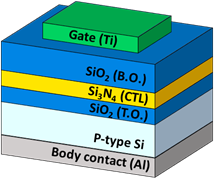Charge migration of 3D NAND flash memories has been studied steadily (7-9). However, these studies indirectly explain lateral charge migration through simulation or electrical characteristic. Therefore, this study proposed a test pattern for charge migration analysis. Using the proposed test pattern, the vertical and lateral charge migration were separated. In addition, we will analyze the mechanism of each charge migration using the separated values.

## II. EXPERIMENT

To fabricate SONOS structure cells for application in non-volatile memory ($NVM$), the 7 nm silicon dioxide ($SiO_{2}$) dielectric for tunneling oxide layer was thermally grown on a prime grade p-type Si substrate with high-purity oxygen gas via a dry oxidation furnace. The silicon nitride ($Si_{3}N_{4}$) as a $CTL$ and the SiO2 blocking oxide layer were deposited by low-pressure chemical vapor deposition ($LPCVD$). The 7 nm Si3N4 $CTL$ and 15 nm blocking oxide layer are considered in this study. In addition, the 100 nm titanium ($Ti$) electrode was deposited for the memory cells. Finally, Al was deposited by RF sputter at back side of silicon wafer for the contact body. Fig. 2 shows the fabricated SONOS capacitor structure.

Fig. 3 shows the gate shape of the test pattern proposed in this study.

Fig. 3(a) shows an antenna-shaped pattern to increase the perimeter length to maximize lateral charge migration. Fig. 3(b) is a mesh-shaped pattern designed to improve process stability and evaluate interference between metal lines compared to antenna-shaped pattern. Each pattern was split in width, distance, area ratio, and perimeter ratio. A square pattern with the same area as the test pattern was additionally designed for reference measurement on each test pattern.

Fig. 3. Gate shape of the proposed test pattern (a) antenna-shaped pattern, (b) mesh-shaped pattern. Here, perimeter of contact pad is much smaller than the total gate perimeter and so the retention characteristics is not affected by the contact pad position.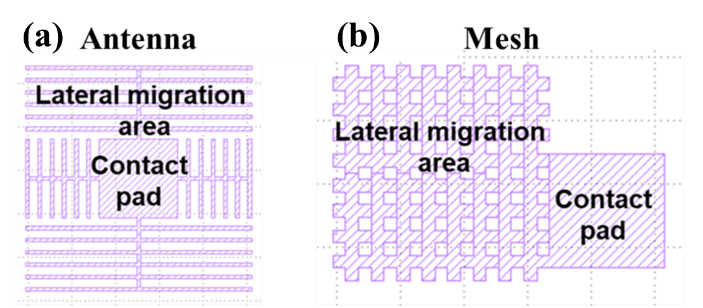Fig. 4. Schematic diagram of electron transfers according to pattern shape (a) test pattern, (b) square pattern. In the square pattern, the lateral charge migration is negligible due to the small perimeter relative to the gate area.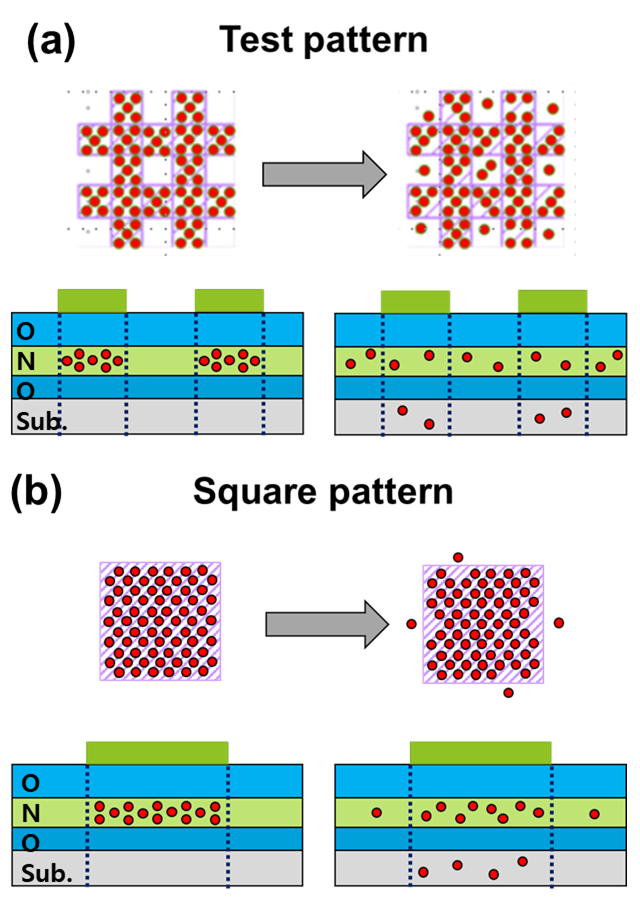Fig. 4 is a schematic diagram showing the movement of electrons in the CTL according to the pattern shape. In case of test pattern, the lateral charge migration is maximized because the perimeter length is designed longer than the square pattern of the same area. Under the same measurement conditions, the charge loss in the square pattern is mostly caused by vertical charge migration. In the test pattern, the charge loss will increase because both vertical and lateral charge migration occur simultaneously. Then, the actual lateral charge migration can be obtained by the difference between the charge loss measurements in the test pattern and the square pattern.

The capacitance-voltage ($C$-$V$) and retention analysis were measured on fabricated capacitors using a Hewlett Packard 4284A precision LCR meter. Retention characteristics for the programmed state were obtained by monitoring the flat band voltage ($V_{FB}$) shift with respect to its initial value and retention tests were performed at room temperature (20 ℃) to 125 ℃.

## III. SIMULATION RESULTS

Fig. 5 shows the $C$-$V$ analysis according to temperature. The same program voltage ($V_{PRG}$) was applied to match the programmed position. After the program, the temperature of the device was increased and the $V_{FB}$ shift was confirmed by measuring the $C$-$V$ at 10 minute intervals from 0 to 20 minutes. The retention measurement shows that the $V_{FB}$ shift to the left over time. At higher temperature, the more $V_{FB}$ shift was observed.

Fig. 6. Charge loss measurement results according to (a) the area ratio in the square pattern, (b) the perimeter ratio in the antenna-shaped pattern.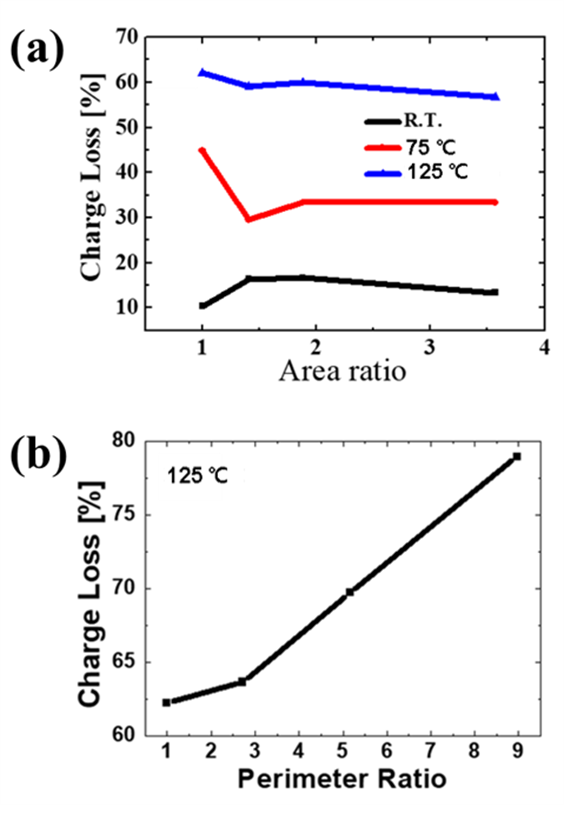$V_{FB}$ was extracted to 80 % of the maximum capacitance. Charge loss is expressed as a percentage and the equation is as follows.

##### (1)
$$\text { Chargeloss }(\%)=\frac{V_{F B} \text { shift }(\text { Retention})}{V_{F B} \text { shift }(P R G)} \times 100$$

Fig. 6 shows the charge loss according to the area ratio in the square pattern and perimeter ratio in the antenna-shaped pattern. The area and perimeter corresponding to "1" are 25764 µm2 and 6344 µm, respectively. In the square pattern, it is assumed that the vertical migration occurs dominantly because the area-to-perimeter is very small. Therefore, Fig. 6(a) shows that the amount of charge loss is almost constant as the area increases. When the temperature was increased, it was confirmed that the amount of charge loss was increased. Fig. 6(b) shows the charge loss measured in the antenna-shaped pattern at a temperature of 125 ℃. As the perimeter increases, the charge loss increases linearly. It can be expected that that the movement of the electron by the lateral charge migration increased with increasing perimeter length.

Fig. 7 shows the charge loss due to the lateral charge migration according to perimeter length at 125 ℃ using the results in Fig. 6. The lateral charge loss was extracted using the difference between Fig. 6(a) and (b).

Fig. 7. Measurement results of the lateral charge loss. according to perimeter length at 125℃.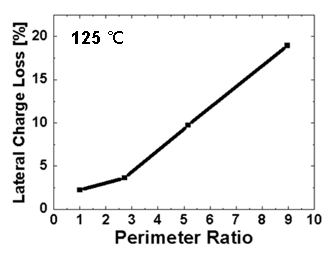Fig. 8. VFB shift results over time in the mesh-shaped pattern.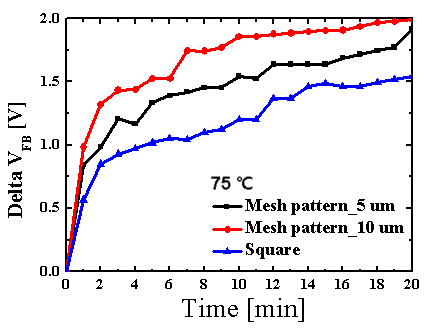In Fig. 7, as perimeter ratio increases, lateral charge loss increases. When the perimeter ratio is small, it is only 5 % of the total charge loss, but when the perimeter ratio is increased to 9, it increases to 25 %.

Fig. 8 shows the $V_{FB}$ shift of the mesh-shaped pattern over time. $V_{FB}$ shift was measured by varying the length of one side of the inner square. As the length of one side increases, the length of the lateral charge migration increases. Therefore, as the length of one side increases, the $V_{FB}$ shift increases. Therefore, the proposed test pattern is very effective for reliability evaluation because it can maximize the lateral charge migration effect.

Fig. 9 shows a graph comparing the effects of lateral and vertical charge loss with decreasing channel length. The effect of lateral charge migration increases as channel length decreases. In this study, the channel length is um level. Because the area of contact with the channel is very large, the vertical charge loss appears larger than lateral charge loss. However, if the channel length is decreased to nm level, lateral charge migration will be the main cause of charge migration (10). Therefore, lateral migration is more important than vertical migration in the reliability problem of 3D SONOS NAND flash memory.

Fig. 9. Comparison of lateral and vertical charge loss according to channel length. Here, the solid line is the result of the reference (10) and the dash line is the result of this study.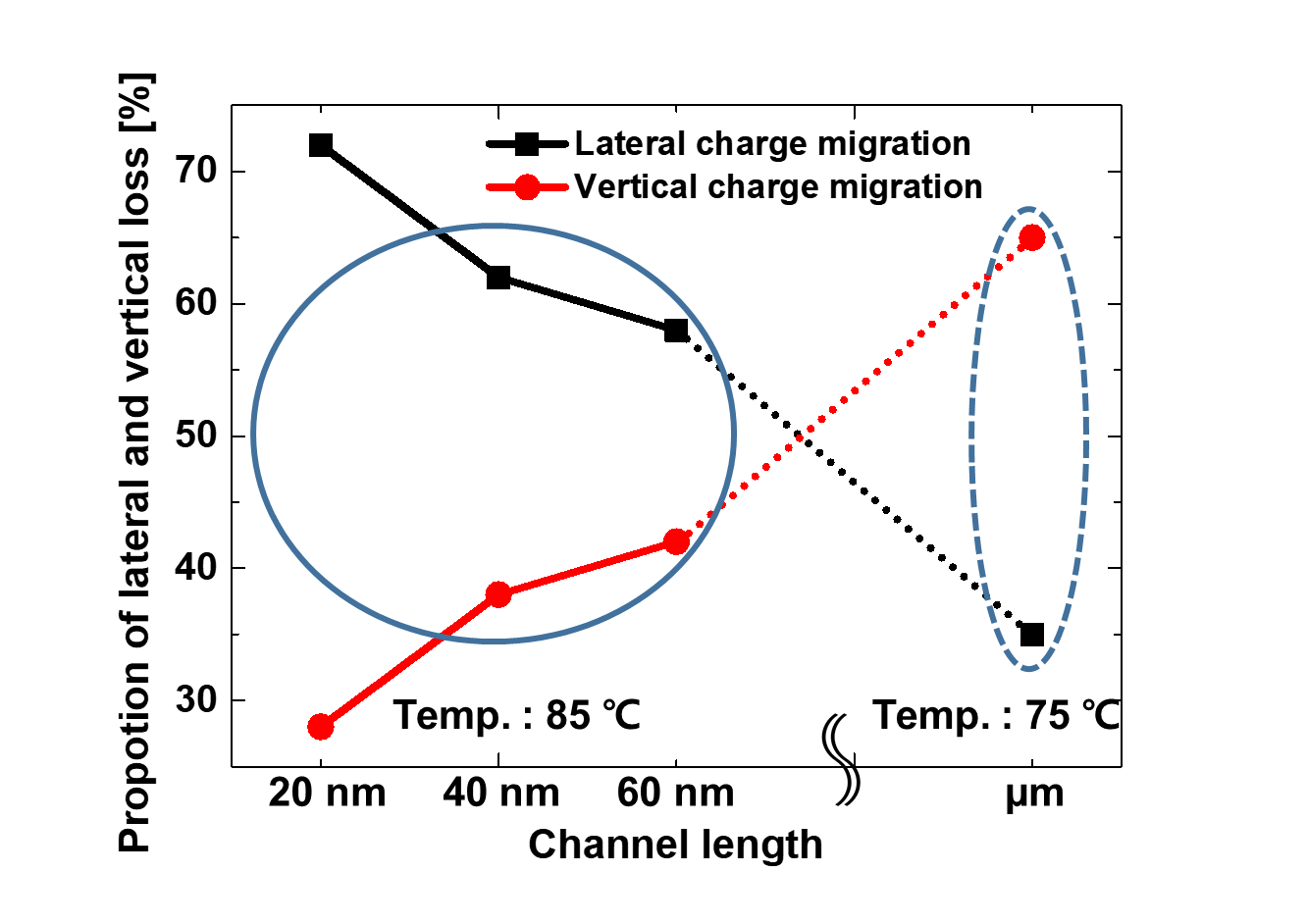Fig. 10. (a) Measurement results of the charge loss in the suggested test pattern and the conventional square pattern according to temperature, (b) redrawn results for fitting to Arrhenius equation to extract the activation energy.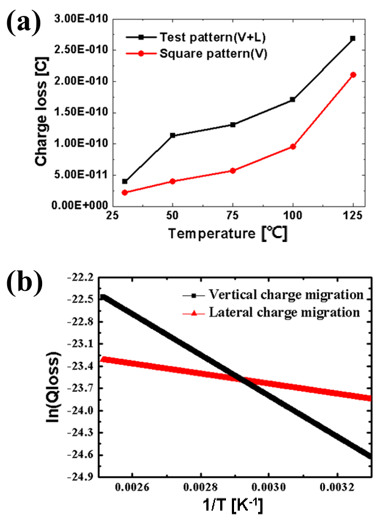To evaluate the activation energy for the charge migration, the temperature dependence of charge loss are measured in antenna-shaped pattern and square pattern as shown in Fig. 10(a), which is redrawn to Fig. 10(b) to apply to the Arrhenius equation. The Arrhenius equation used is as follows.

Fig. 11. Modeling diagram of lateral charge migration by conduction-band diffusion. In the elevated temperature condition, more conduction electrons can be observed especially in the Si3N4. This is because the electrons in the shallow trap that participate in conduction-band diffusion increase by heat. Considering that the distance between the bandgap trap is not so close in the insulator, the probability of lateral diffusion by hopping will be much lower.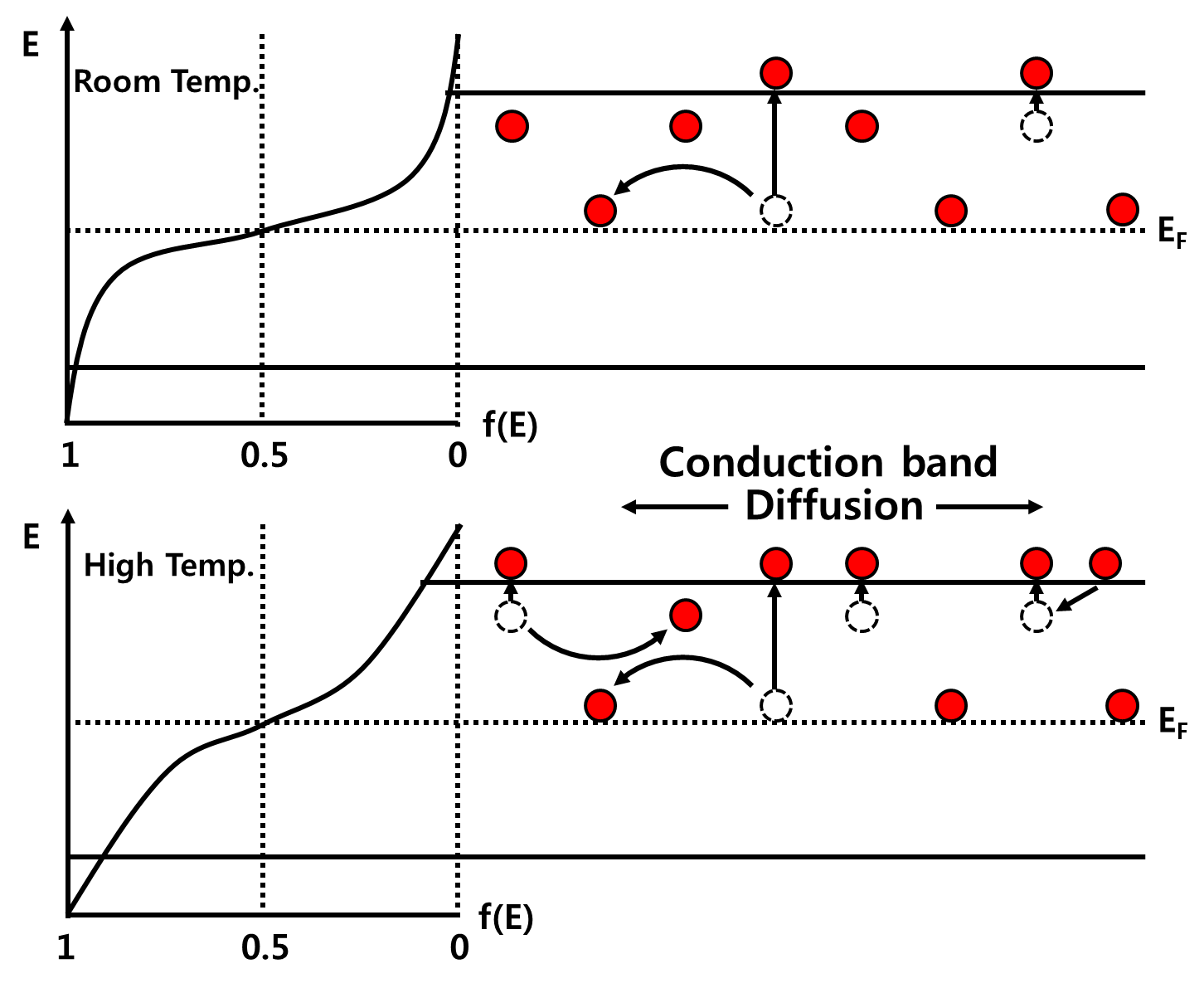##### (2)
$$Q=Q_{0} \exp \left(\frac{-E_{A}}{k T}\right)$$

##### (3)
$$\ln Q=\left(\frac{-E_{A}}{k} \frac{1}{T}\right)+\ln Q_{0}$$

The activation energy in the square pattern ($E_{A,vertical}$) where the vertical migration is main factor for the charge loss was calculated as 0.238 eV. And the activation energy in the test pattern ($E_{A,Lateral}$) where only the lateral migration is considered by subtracting the vertical charge loss was calculated as 0.058 eV. It is noticeable that $E_{A,Lateral}$ is very smaller than $E_{A,vertical}$ and similar results have been reported based on the simulation by other research group (11,12). This is because lateral charge migration occurs above the conduction band. The electrons in the shallow traps move up the conduction band by heat, and the electrons in the conduction band can move easily. Therefore, it can be expected for electron in the conduction to diffuse with a relatively small energy, which is illustrated in Fig. 11 by the lateral charge migration by the conduction band diffusion.

## IV. CONCLUSIONS

In this study, we proposed a test pattern for analyzing the charge migration in 3D SONOS flash memory. For the analysis, a capacitor with ONO structure was fabricated, and C-V curve and retention analysis were performed according to the temperature. Vertical charge loss was measured by retention measurement in the conventional square pattern, and total charge loss was measured by increasing the perimeter ratio using the test pattern. And, substantial lateral charge losses were extracted using the difference between the square pattern and the test pattern. In addition, the activation energy of lateral and vertical charge migration was extracted using the Arrhenius equation. From the extracted activation energy, lateral charge migration is modeled by the conduction band diffusion. Because the suggested test pattern can measure each value for the lateral and vertical migration occurring in the 3D SONOS flash memory, it is expected that a clear analysis of the reliability problem that can be caused by the charge migration is possible.

### ACKNOWLEDGMENTS

This research was supported by Nano·Material Technology Development Program through the National Research Foundation of Korea(NRF) funded by the Ministry of Science, ICT and Future Planning (2009-0082580), and by a National Research Foundation of Korea (NRF) grant, funded by the Korea government (MSIP) (NRF-2019M3F3A1A01074448)

### REFERENCES

1
Shibata N., Kanda K., Shimizu T., Nakai J., 2019, 13.1 A 1.33 Tb 4-bit/Cell 3D-Flash Memory on a 96-Word-Line-Layer Technology., IEEE Inter-national Solid-State Circuits Conference-(ISSCC). IEEE, pp. 210-2122
Rizk R., Rizk D., Kumar A., Bayoumi M., 2019, Demystifying Emerging Nonvolatile Memory Technologies: Understanding Advantages, Challenges, Trends, and Novel Applications., 2019 IEEE International Symposium on Circuits and Systems (ISCAS). IEEE, pp. 1-53
Jeong W., Im J. W., 2015, A 128 Gb 3b/cell V-NAND flash memory with 1 Gb/s I/O rate., IEEE Journal of Solid-State Circuits, Vol. 51, pp. 204-2124
Maejima H., Kanda K., Fujimura S., Takagiwa T., 2018, A 512Gb 3b/Cell 3D flash memory on a 96-word-line-layer technology., 2018 IEEE International Solid-State Circuits Conference-(ISSCC). IEEE, pp. 336-3385
Maconi A., Arreghini A., Compagnoni C. M., V G., den bosch , Spinelli A. S., Houdt J. V., Lacaita A. L., 2012, Comprehensive investigation of the impact of lateral charge migration on retention performance of planar and 3D SONOS devices., Solid-State Electronics, Vol. 74, pp. 64-706
Hsiao W. H., Wang N. J., Lee M. Y., Kuo L. K., 2019, Modeling of Apparent Activation Energy and Lifetime Estimation for Retention of 3D SGVC Memory., 2019 IEEE International Reliability Physics Symposium (IRPS). IEEE, pp. 1-57
Kim S. H., Yang S. D., Kim J. S., Jeong J. K., Lee H. D., W Lee G., Aug 2015, Charge Spreading Effect of Stored Charge on Retention Characteristics in SONOS NAND Flash Memory Devices, Trans. Electr. Electron. Mater., Vol. 16, pp. 183-1868
Maconia A., Arreghini A., Monzio Compagnoni C., Van den bosch G., Spinelli A. S., Van Houdt J., Lacaita A. L., Aug 2012, Comprehensive investigation of the impact of lateral charge migration on retention performance of planar and 3D SONOS devices, Solid-State Electronics, Vol. 74, pp. 64-709
Liu Y. H., Jiang C. M., Chen W. C., Wang T., Tsai W. J., Lu T. C., Chen K. C., Lu C. Y., Jan 2017, Electric Field Induced Nitride Trapped Charge Lateral Migration in a SONOS Flash Memory, IEEE Electron Device Lett., Vol. 38, pp. 48-5110
Grossi A., Zambelli C., Olivo P., 2016, Reliability of 3D NAND flash memories., 3D Flash Memories. Springer, Dordrecht, pp. 29-6211
Lee K. H., Kang M. G., Hwang Y. C., 2015, Modeling of apparent activation energy and lifetime estimation in NAND flash memory., Semiconductor Science and Technology, Vol. 30, pp. 12500612
Woo C. B., Lee M. W., Kim S. k., Park J. Y., 2019, Modeling of Charge Loss Mechanisms during the Short Term Retention Operation in 3-D NAND Flash Memories., 2019 Symposium on VLSI Technology. IEEE, pp. T214-T215## Author

received a B.S. degree in electronic engineering in 2016 and is currently working toward an integrated Ph.D. program in the Department of Electronics Engi-neering from the Chungnam National University, Daejeon, Korea.

His research interests include flash memory, oxide thin film transistor.

is currently studying toward an B.S. degree in the Department of Electronics Engi-neering from the Chungnam National University, Daejeon, Korea.

His research interests include reliability and analysis of flash memory.

is currently studying toward an B.S. degree in the Department of Electronics Engi-neering from the Chungnam National University, Daejeon, Korea.

His research interests include reliability and analysis of flash memory.

received B.S., M.S., and Ph.D. degrees from Korea Advanced Institute of Science and Technology (KAIST), Daejeon, Korea, in 1990, 1992, and 1996, respectively, all in electrical engi-neering.

In 1993, he joined LG Semicon Co., Ltd. (currently SK hynix Semiconductor), Cheongju, Korea, where he was involved in the development of 0.35-, 0.25-, and 0.18-μm CMOS technologies, respectively.

He was also responsible for the development of 0.15- and 0.13-μm CMOS technologies.

Since 2001, he has been with Chungnam National University, Daejeon, and now is Professor with the Department of Electronics Engineering.

From 2006 to 2008, he was with the University of Texas, Austin, and SEMATECH, Austin, as a Visiting Scholar.

His research interests are nanoscale CMOS technology and its reliability physics, silicide technology, and test element group design.

His research interests also include sensitivity improvement of sensors, and development of high performance sensors.

Dr. Lee is a member of the Institute of Electronics Engineers of Korea.

He received the Excellent Professor Award from Chungnam National University in 2001, 2003 and 2014.

received B.S., M.S., and Ph.D. degrees in Electrical Engineering from Korea Advanced Institute of Science and Technology (KAIST), Daejeon, Korea, in 1994, 1996, and 1999, respectively.

In 1999, she joined Hynix Semiconductor Ltd. (currently SK Hynix Semiconductor Ltd.) as a senior research engineer, where she was involved in the development of 0.115-Se and 0.09—S DDR II DRAM technologies.

Since 2005, she has been at Chungnam National University, Daejeon, Korea, as a Professor with the Department of Electronics Engineering.

Her main research fields are flash memory and flexible display technology including fabrication, electrical analysis, and modeling.